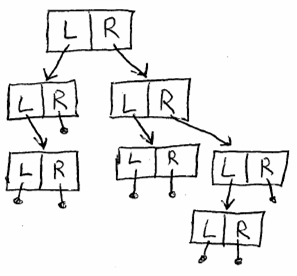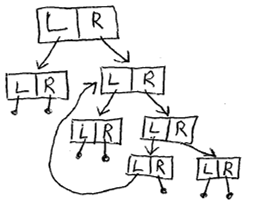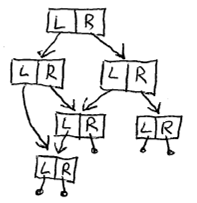# Immutable Trees and Threading Evil - Part 1

I was reading a post in Eric Lippert's Blog and started to post a reply, but when I realized just how much I needed to say, I decided it should be it's own post, nay: series of posts on my blog. Let me take you on an interesting tour of immutable data structures (good!) and threading behavior (scary!).

First, we are going to create an immutable binary tree type. A binary tree looks something like the structure shown in the diagram.Typically, there is data in the individual nodes, but I am just interested in the structure of the tree so I'll leave out the data. Immutable means that the nodes cannot be modified once created—normally the child nodes are passed into the constructor. Here is the code in Java for a simple immutable binary tree.

```/** An immutable binary tree (with no values in it, making it fairly useless). */
public class TreeNode {
private TreeNode left;
private TreeNode right;
/** Constructor takes a left and right child or a null if either is empty. */
public TreeNode(TreeNode left, TreeNode right) {
this.left = left;
this.right = right;
}
public TreeNode getLeft() { return left; }
public TreeNode getRight() { return right; }
}
```

"But Michael", you say, "you could have declared 'left' and 'right' to be final." Yes, I know... and I'll get back to that later.

Now, one of the nice features of immutable trees (at least according to Eric's post) is supposed to be that they are guaranteed to be tree-shaped: they never contain a loop. I am going to show that this isn't necessarily true. We'll start by creating a RandomTreeBuilder that builds trees randomly, mixing in nodes from a NodePool. First, the NodePool:

```/** A class storing some TreeNode instances. */
public class NodePool {
final int POOL_SIZE = 100;
TreeNode[] nodes = new TreeNode[POOL_SIZE];
/** Obtains a random node from the pool. */
public TreeNode takeRandomNode() {
return nodes[((int) (POOL_SIZE * Math.random()))];
}
/** Puts a new node into the pool. */
public void putNode(TreeNode node) {
nodes[((int) (POOL_SIZE * Math.random()))] = node;
}
}
```

Notice that this is not designed with any locking. Java guarantees us that the references will be written correctly—whether or not that makes it "threadsafe" depends on just what you think the word means. Now we'll see the RandomTreeBuilder. It does just what its name suggests:

```/** A Runnable that builds trees randomly. */
public class RandomTreeBuilder implements Runnable {
public final static int NUM_NODES_PER_THREAD = 10000;

private NodePool nodePool;
/** Constructor; sets nodePool. */
public RandomTreeBuilder(NodePool nodePool) {
this.nodePool = nodePool;
}

public void run() {
for (int i=0; i<NUM_NODES_PER_THREAD; i++) {
TreeNode leftChild = nodePool.takeRandomNode();
TreeNode rightChild = nodePool.takeRandomNode();
nodePool.putNode(new TreeNode(leftChild, rightChild));
}
}
}
```

So we have something which will mix and match, grabbing a node here and a node there and build up a tree from it. We could run one RandomTreeBuilder or even several of them in different threads. The question is whether it could ever create a loop. A tree with a loop looks like this diagram:A node can appear in a tree multiple times without creating a loop, as shown in this diagram:So a node that is duplicated within a tree isn't a problem, but a node which has one of its parents as a descendant is. Loops are bad because they cause us to get into infinite loops (or infinite recursion) when we attempt to traverse the tree. Here is some code that looks for loops:

```/** Class used to check a TreeNode for loops. */
public class LoopSearcher {
private java.util.Set loopFreeNodes = new java.util.HashSet();

/** Tests whether a node contains a loop. */
public boolean hasLoop(TreeNode node) {
return hasLoop(node, new java.util.HashSet<TreeNode>());
}

/**
* Used to search for loops in a tree. Returns true if the given
* node contains a loop, or if it or a decendent appears in the
* 'parents' set. If it returns false, then the 'parents' set
* is unmodified.
*/
private boolean hasLoop(TreeNode node, java.util.Set<TreeNode> parents) {
if (node == null) {
return false; // wasn't even an actual node
} else if (loopFreeNodes.contains(node)) {
return false; // this node was already proven loop-free
} else if (parents.contains(node)) {
return true; // WOOT! We found a loop!!!
} else {
// Recursively search our children
if (hasLoop(node.getLeft(), parents)) return true;
if (hasLoop(node.getRight(), parents)) return true;
parents.remove(node);

// Searched but didn't find a loop.
return false;
}
}
}
```

Notice the use of a cache of nodes known not to contain loops: without this detail the algorithm would be unacceptably slow.

So now we have all of the pieces in place, let's write a Main program that puts them together. We'll run several RandomTreeBuilders at once in different threads and at the end check whether any loops were created:

```/** Main() and methods needed for this demonstration. */
public class Main {
public final static int NUM_THREADS = 6;

public static void main(String[] args) {
// -- Make a NodePool --
final NodePool nodePool = new NodePool();

// -- Create worker threads to make random trees --
for (int i=0; i<NUM_THREADS; i++) {
}

// -- Wait for all the worker threads to finish --
try {
} catch(InterruptedException err) {
// Ignore it.
}
}

// -- See if loops were created --
LoopSearcher loopSearcher = new LoopSearcher();
for (TreeNode node : nodePool.nodes) {
if (loopSearcher.hasLoop(node)) {
System.out.println("We Found A Loop!!");
return;
}
}
System.out.println("No loop found.");
}
}
```

Now... the question at hand is whether this can ever print out "We Found A Loop!!". You would think the answer was "No", because the nodes are immutable (so they don't change after creation) and the child nodes are passed in the constructor (so they have to have been created before the parent). But I maintain that it CAN create a loop, and in Part 2 I will try to explain how.

Posted Wed 19 December 2007 by mcherm in Programming